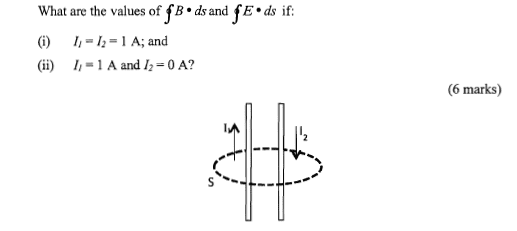# Two long wires carrying current

## Homework Statement

Two long wires carrying current I1 and I2 in opposite directions pass through a closed path S as shown in the picture.
What are the values of ∫B⋅ds and ∫E⋅ds if:
i) I1=I2=1A
ii) I1=1A and I2=0A?## Homework Equations

Ampere's Law: ∫B⋅ds=μ0I

## The Attempt at a Solution

For i, ∫B⋅ds=μ0(I1-I2)=μ0(0)=0
For ii, ∫B⋅ds=μ0(I1)=μ0(1)=μ0

Is these correct? But I have no idea of finding ∫E⋅ds

BvU
Homework Helper
What about the righthand side if you knw the currents are constant ?

rude man
Homework Helper
Gold Member